Video Tutorial Moles - Mass

Quick Notes Moles from Mass

• The number of moles in a sample can be calculated using the formula: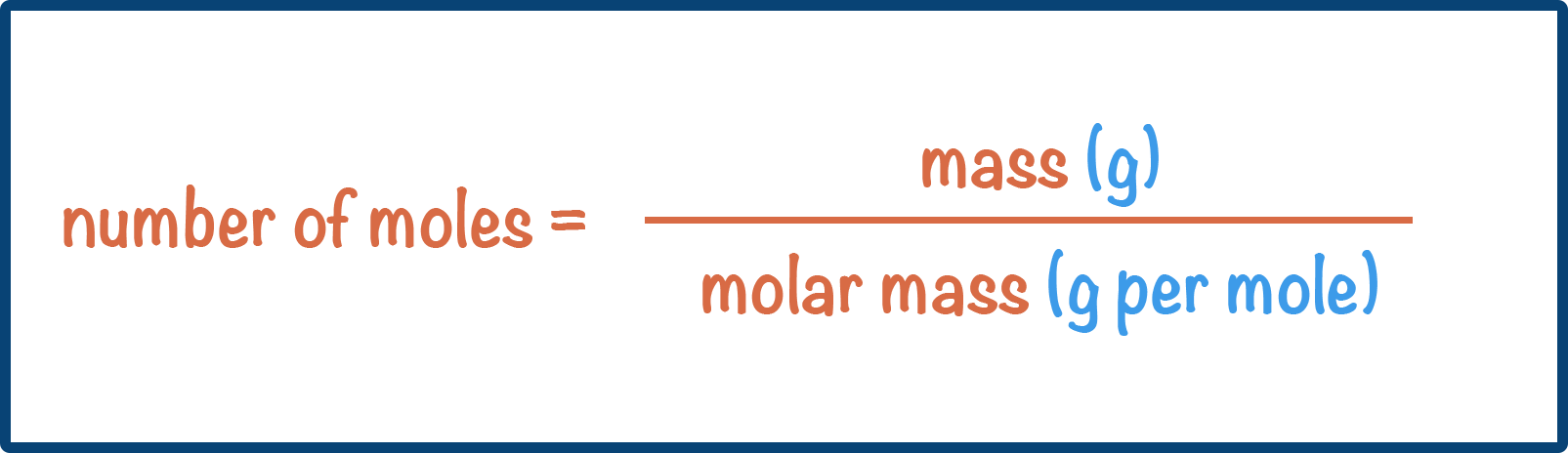• The value of relative atomic mass of an element = value of molar mass (in grams per mol).
• The value of relative formula and molecular mass = value of molar mass (in grams per mol).
• This means moles can be calculated using:Full Notes Moles from Mass

There are two main ways of measuring how much of a substance you have - mass and volume.

We know atoms of different elements have different relative atomic masses (). The relative atomic mass of an element is numerically the same as the molar mass of the element (mass of one mole). For example, the relative mass of one sodium atom is 23; the mass of 1 mole of sodium atoms is 23g.

As the values for molar mass and relative atomic mass are the same, it is usually easier to just refer to ‘relative atomic mass’ when calculating moles (as we will on this page).

## Calculating Moles

Let’s take oxygen. Oxygen has a relative atomic mass of 16. This means, 1 mole of oxygen atoms has a mass of 16g.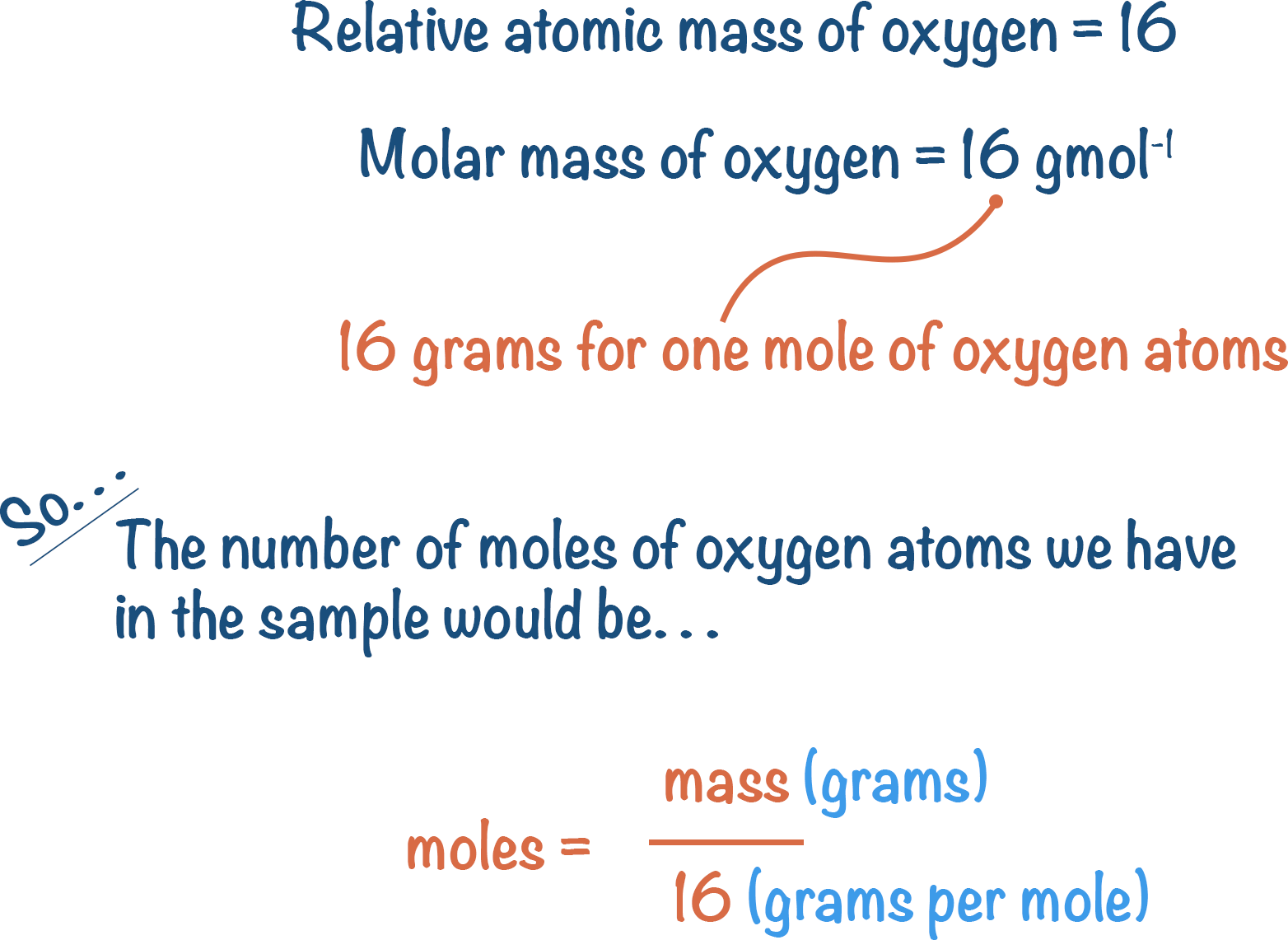Let’s check: if we have 16g of oxygen, how many moles do we have?

We need to know the mass (in this case, 16g) and we can look-up the relative atomic mass of oxygen in the periodic table to know the molar mass (16 g per mole).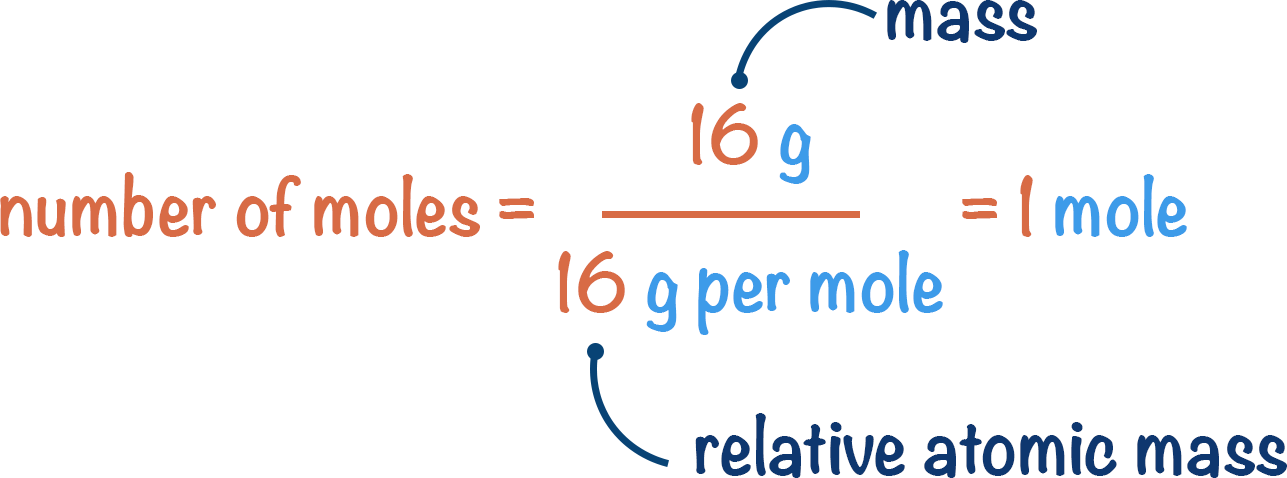number of moles = 16g / 16g per mole = 1 mole

This gives us the following equation: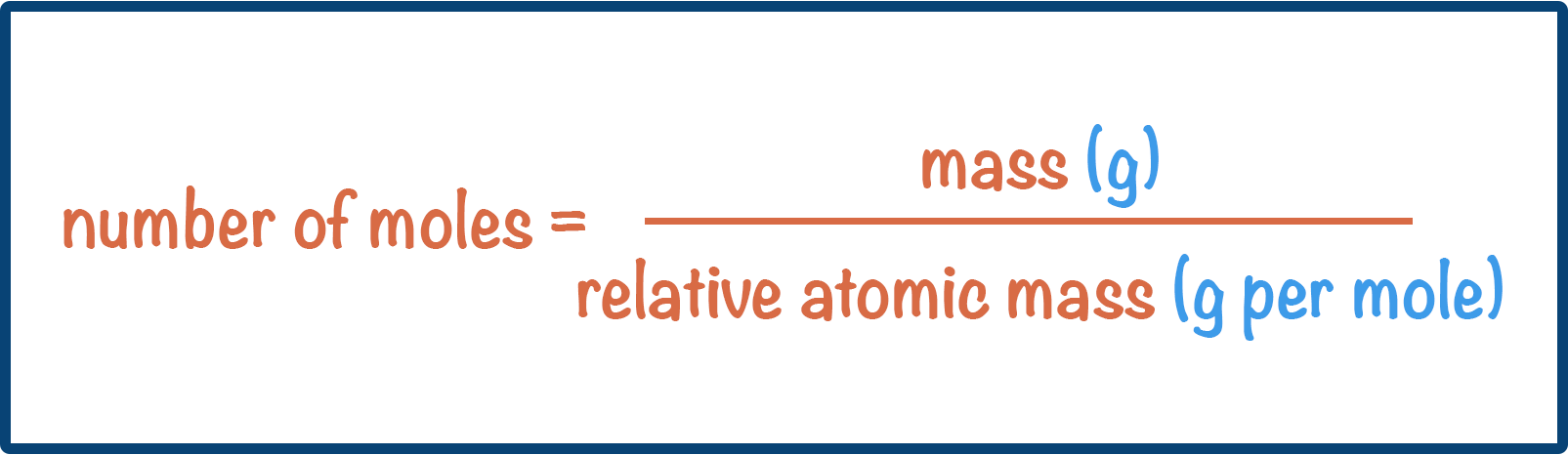You may have spotted a problem. Oxygen does not actually exist as lone atoms – oxygen is found naturally in the form O2.

O2 is a molecule, not an atom. So, we cannot use relative atomic mass to calculate the number of moles in a given mass. Instead, we need to use relative molecular mass.

Relative molecular mass is the relative atomic masses of all the atoms within a molecule added together.

In one molecule of oxygen (O2) we have two atoms of oxygen. The relative atomic mass of oxygen is 16. So, for two atoms the relative molecular mass will be 16 + 16 = 32. This means the molar mass would be 32g mol-1.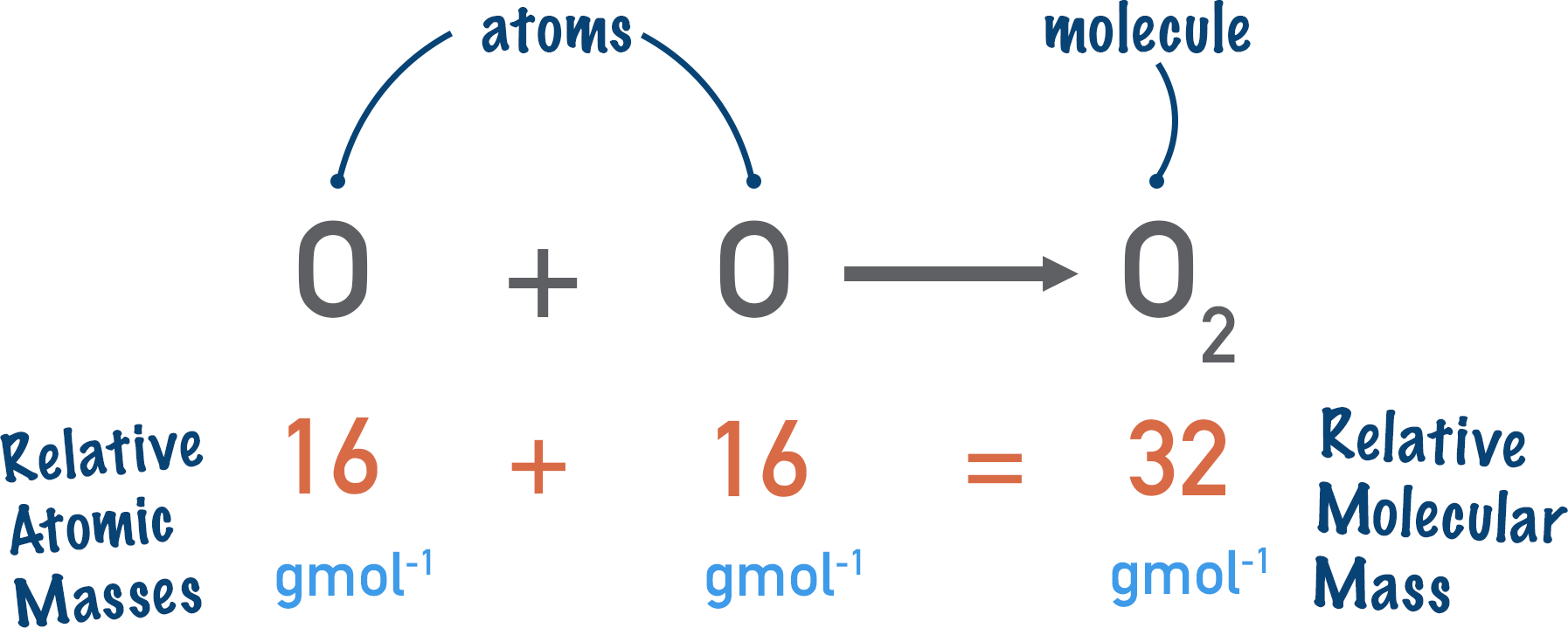So, if we have 16 grams of oxygen gas. How many moles of oxygen molecules (O2) would we have?

Remember the equation:mass of O2 = 16 grams

relative molecular mass = 16 + 16 = 32 grams per mole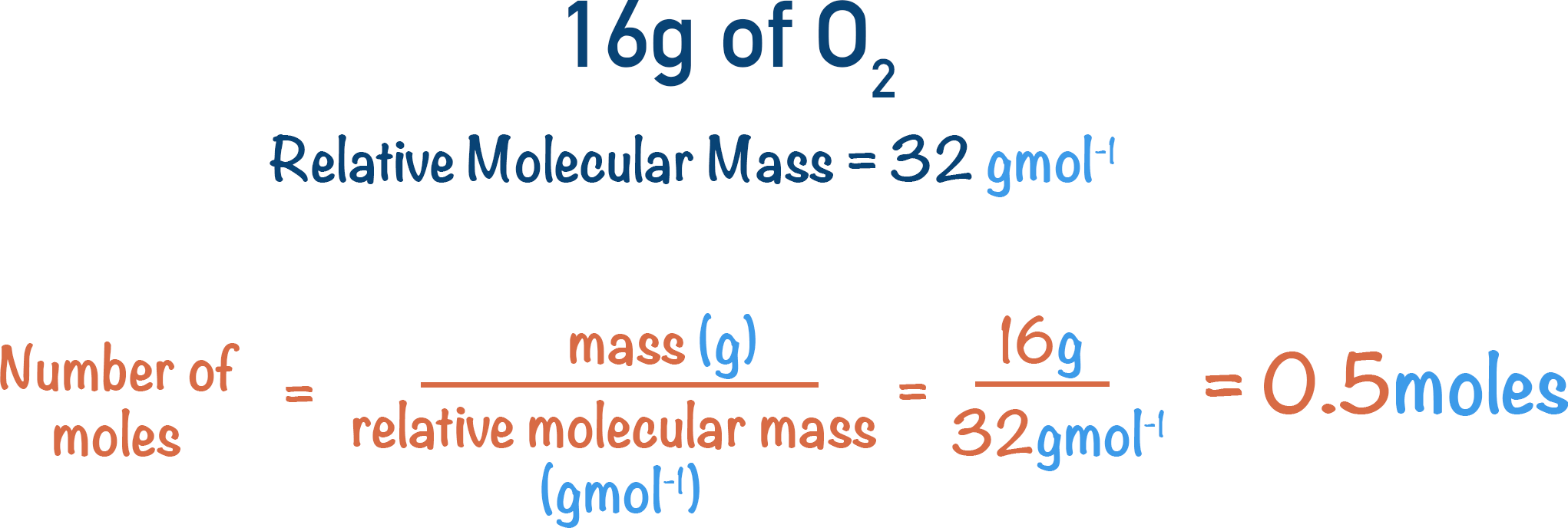This means we have 0.5 moles of O2 molecules in 16 grams of oxygen gas. Since one molecule is made of two oxygen atoms, the number of oxygen (O) atoms would be 0.5 x 2 = 1 mole. The number of oxygen atoms in 16 grams of oxygen gas would simply be 1 mole.